Test: Discrete Time Signal Analysis - 1

# Test: Discrete Time Signal Analysis - 1

Test Description

## 15 Questions MCQ Test Digital Signal Processing | Test: Discrete Time Signal Analysis - 1

Test: Discrete Time Signal Analysis - 1 for Electrical Engineering (EE) 2022 is part of Digital Signal Processing preparation. The Test: Discrete Time Signal Analysis - 1 questions and answers have been prepared according to the Electrical Engineering (EE) exam syllabus.The Test: Discrete Time Signal Analysis - 1 MCQs are made for Electrical Engineering (EE) 2022 Exam. Find important definitions, questions, notes, meanings, examples, exercises, MCQs and online tests for Test: Discrete Time Signal Analysis - 1 below.
Solutions of Test: Discrete Time Signal Analysis - 1 questions in English are available as part of our Digital Signal Processing for Electrical Engineering (EE) & Test: Discrete Time Signal Analysis - 1 solutions in Hindi for Digital Signal Processing course. Download more important topics, notes, lectures and mock test series for Electrical Engineering (EE) Exam by signing up for free. Attempt Test: Discrete Time Signal Analysis - 1 | 15 questions in 15 minutes | Mock test for Electrical Engineering (EE) preparation | Free important questions MCQ to study Digital Signal Processing for Electrical Engineering (EE) Exam | Download free PDF with solutions
 1 Crore+ students have signed up on EduRev. Have you?
Test: Discrete Time Signal Analysis - 1 - Question 1

### What is the Fourier series representation of a signal x(n) whose period is N?

Detailed Solution for Test: Discrete Time Signal Analysis - 1 - Question 1

Explanation: Here, the frequency F0 of a continuous time signal is divided into 2π/N intervals.
So, the Fourier series representation of a discrete time signal with period N is given as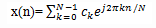where ck is the Fourier series coefficient

Test: Discrete Time Signal Analysis - 1 - Question 2

### What is the expression for Fourier series coefficient ck in terms of the discrete signal x(n)?

Detailed Solution for Test: Discrete Time Signal Analysis - 1 - Question 2

Explanation: We know that, the Fourier series representation of a discrete signal x(n) is given as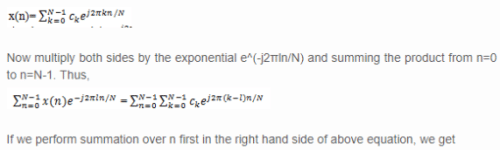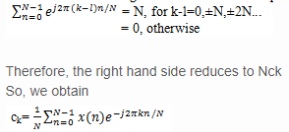Test: Discrete Time Signal Analysis - 1 - Question 3

### Which of the following represents the phase associated with the frequency component of discrete-time Fourier series(DTFS)?

Detailed Solution for Test: Discrete Time Signal Analysis - 1 - Question 3

Explanation: We know that,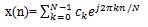In the above equation, ck represents the amplitude and ej2πkn/N represents the phase associated with the frequency component of DTFS.

Test: Discrete Time Signal Analysis - 1 - Question 4

The Fourier series for the signal x(n)=cos√2πn exists.

Detailed Solution for Test: Discrete Time Signal Analysis - 1 - Question 4

Explanation: For ω0=√2π, we have f0=1/√2. Since f0 is not a rational number, the signal is not periodic. Consequently, this signal cannot be expanded in a Fourier series.

Test: Discrete Time Signal Analysis - 1 - Question 5

What are the Fourier series coefficients for the signal x(n)=cosπn/3?

Detailed Solution for Test: Discrete Time Signal Analysis - 1 - Question 5

Explanation: In this case, f0=1/6 and hence x(n) is periodic with fundamental period N=6.
Given signal is x(n)= cosπn/3=cos2πn/6=1/2 e^(j2πn/6)+1/2 e^(-j2πn/6)
We know that -2π/6=2π-2π/6=10π/6=5(2π/6)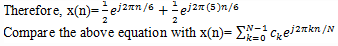So, we get c1=c2=c3=c4=0 and c1=c5=1/2.

Test: Discrete Time Signal Analysis - 1 - Question 6

What is the Fourier series representation of a signal x(n) whose period is N?

Detailed Solution for Test: Discrete Time Signal Analysis - 1 - Question 6

Explanation: Here, the frequency F0 of a continuous time signal is divided into 2π/N intervals.
So, the Fourier series representation of a discrete time signal with period N is given aswhere ck is the Fourier series coefficient

Test: Discrete Time Signal Analysis - 1 - Question 7

What is the average power of the discrete time periodic signal x(n) with period N ?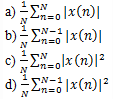Detailed Solution for Test: Discrete Time Signal Analysis - 1 - Question 7

Explanation: Let us consider a discrete time periodic signal x(n) with period N.
The average power of that signal is given asTest: Discrete Time Signal Analysis - 1 - Question 8

What is the equation for average power of discrete time periodic signal x(n) with period N in terms of Fourier series coefficient ck?

Detailed Solution for Test: Discrete Time Signal Analysis - 1 - Question 8

Explanation: We know that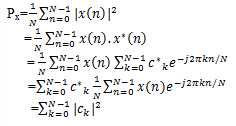Test: Discrete Time Signal Analysis - 1 - Question 9

What is the Fourier transform X(ω) of a finite energy discrete time signal x(n)?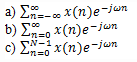d) None of the mentioned

Detailed Solution for Test: Discrete Time Signal Analysis - 1 - Question 9

Explanation: If we consider a signal x(n) which is discrete in nature and has finite energy, then the Fourier transform of that signal is given as

Test: Discrete Time Signal Analysis - 1 - Question 10

What is the period of the Fourier transform X(ω) of the signal x(n)?

Detailed Solution for Test: Discrete Time Signal Analysis - 1 - Question 10

Explanation: Let X(ω) be the Fourier transform of a discrete time signal x(n) which is given as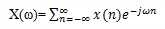Now
So, the Fourier transform of a discrete time finite energy signal is periodic with period 2π.

Test: Discrete Time Signal Analysis - 1 - Question 11

What is the synthesis equation of the discrete time signal x(n), whose Fourier transform is X(ω)?

Detailed Solution for Test: Discrete Time Signal Analysis - 1 - Question 11

Explanation: We know that the Fourier transform of the discrete time signal x(n) is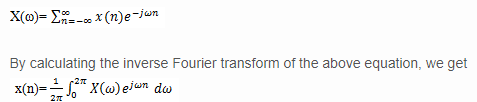The above equation is known as synthesis equation or inverse transform equation.

Test: Discrete Time Signal Analysis - 1 - Question 12

What is the value of discrete time signal x(n) at n=0 whose Fourier transform is represented as below?

Detailed Solution for Test: Discrete Time Signal Analysis - 1 - Question 12

Explanation: We know that,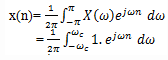At n = 0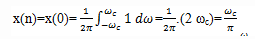Therefore, the value of the signal x(n) at n=0 is ω_c/π.

Test: Discrete Time Signal Analysis - 1 - Question 13

What is the value of discrete time signal x(n) at n≠0 whose Fourier transform is represented as below?

Detailed Solution for Test: Discrete Time Signal Analysis - 1 - Question 13

Explanation: We know that,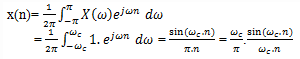Test: Discrete Time Signal Analysis - 1 - Question 14

The oscillatory behavior of the approximation of XN(ω) to the function X(ω) at a point of discontinuity of X(ω) is known as Gibbs phenomenon.

Detailed Solution for Test: Discrete Time Signal Analysis - 1 - Question 14

Explanation: We note that there is a significant oscillatory overshoot at ω=ωc, independent of the value of N. As N increases, the oscillations become more rapid, but the size of the ripple remains the same. One can show that as N→∞, the oscillations converge to the point of the discontinuity at ω=ωc. The oscillatory behavior of the approximation of XN(ω) to the function X(ω) at a point of discontinuity of X(ω) is known as Gibbs phenomenon.

Test: Discrete Time Signal Analysis - 1 - Question 15

What is the energy of a discrete time signal in terms of X(ω)?

Detailed Solution for Test: Discrete Time Signal Analysis - 1 - Question 15

Explanation: We know that,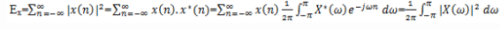## Digital Signal Processing

3 videos|50 docs|54 tests
 Use Code STAYHOME200 and get INR 200 additional OFF Use Coupon Code
Information about Test: Discrete Time Signal Analysis - 1 Page
In this test you can find the Exam questions for Test: Discrete Time Signal Analysis - 1 solved & explained in the simplest way possible. Besides giving Questions and answers for Test: Discrete Time Signal Analysis - 1, EduRev gives you an ample number of Online tests for practice

## Digital Signal Processing

3 videos|50 docs|54 tests